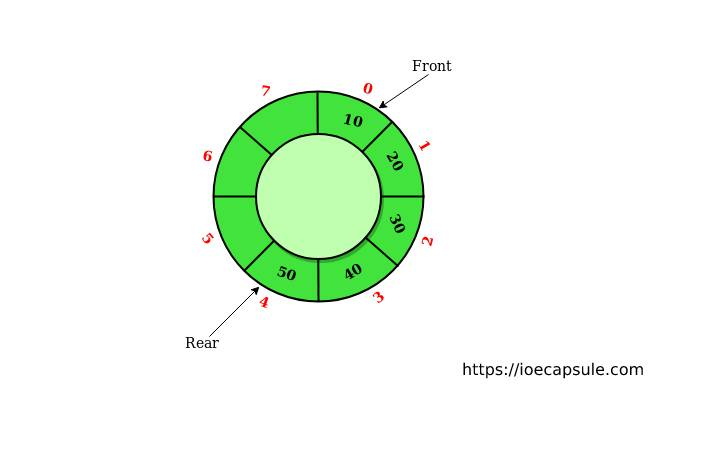### Queue: Circular Queue ImplementationData Structures

A Queue is a linear, ordered collection of items or data structure in which the operations are performed in First In First Out fashion . Meaning, it is always the first item to be put into the queue, and first item is to be removed first .

In the previous article, we discussed about linear queue and also we implemented linear queue using c++. If you haven’t gotten into that, check that out.

Queue: Template Implementation Of Dynamic Linear Queue Using C++

#### Problems With Linear Queue

Both the rear and front indices are increase but never decreased. As items are removed from the queue, the storage space at the beginning of the array is discarded and never used again. Wastage of the space is the main problem with linear queue.

In this article, we are going to talk about circular queue, which is going to remove the limitation of linear queue, mentioned above.

#### What is Circular Queue ?

It is the circular array implementation in which we view array as circle rather than linear . In this implementation, the first element of array is immediately followed by its last element. By increment either rear or front index, we can always insert an item unless the array is fully occupied.

#### Algorithm For Insertion

Initially,

``````
front = 0
rear = 0``````

1.Start

2. Check if queue is full or not

``````
if((rear + 1) % QUEUE_SIZE) = front)
print "queue is full"``````

3.Otherwise,

``````
rear = (rear + 1) % QUEUE_SIZE
read data and store in rear position``````

4.Stop

#### Algorithm For Deletion

1.Start

2.Check if queue is empty or not

``````
if(rear = front)
print "queue is full"``````

3.Otherwise,

``````
front = (front + 1) % QUEUE_SIZE``````

4.Stop

#### What’s Next ?

Queue: Template Implementation Circular Queue Using C++